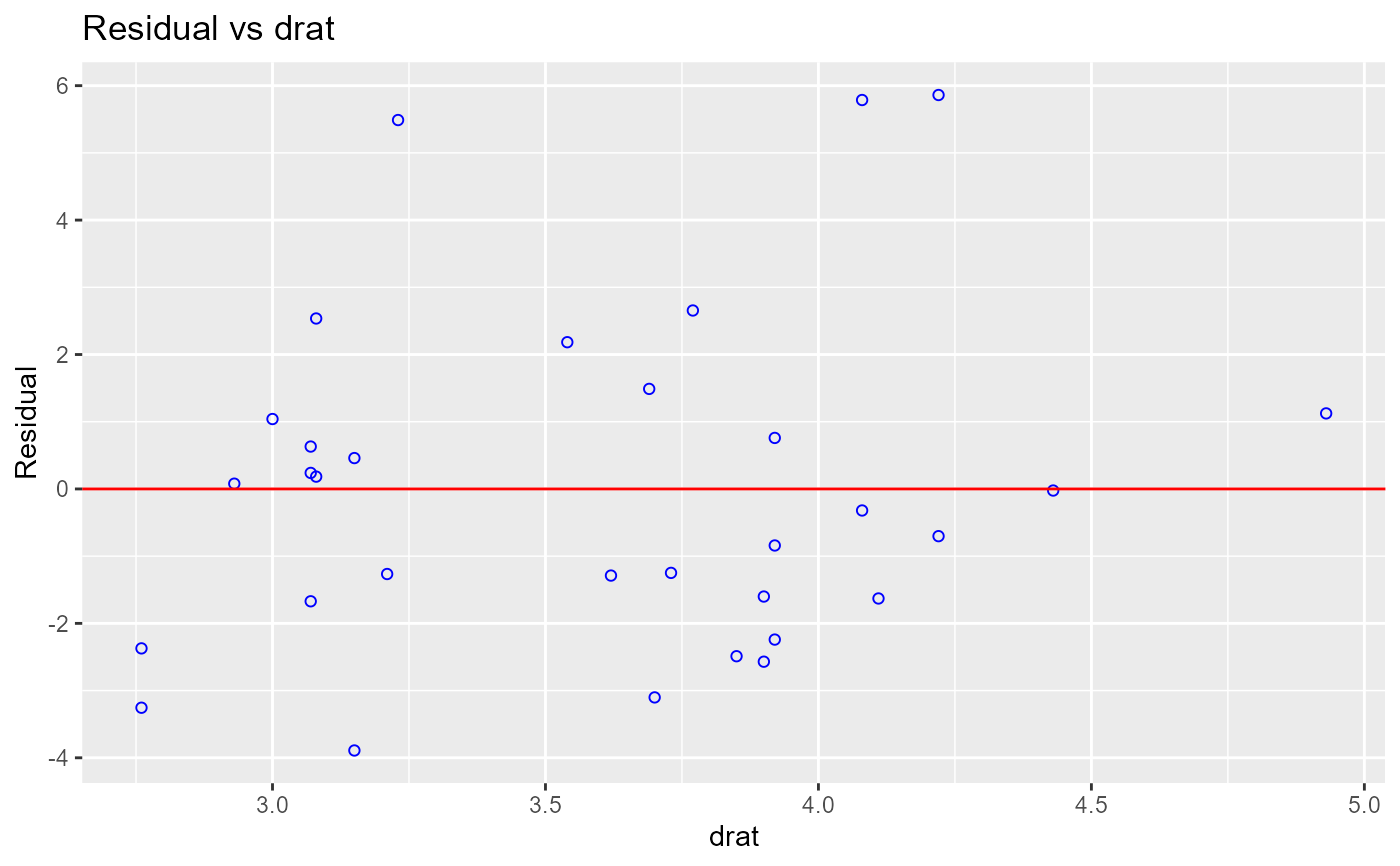Graph to determine whether we should add a new predictor to the model already containing other predictors. The residuals from the model is regressed on the new predictor and if the plot shows non random pattern, you should consider adding the new predictor to the model.

ols_plot_resid_regressor(model, variable, print_plot = TRUE)

## Arguments

model An object of class lm. New predictor to be added to the model. logical; if TRUE, prints the plot else returns a plot object.

## Deprecated Function

ols_rvsr_plot() has been deprecated. Instead use ols_plot_resid_regressor().

model <- lm(mpg ~ disp + hp + wt, data = mtcars)
ols_plot_resid_regressor(model, 'drat')